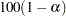EXACT Statement

EXACT statistic-options </ computation-options> ;
The EXACT statement requests exact tests for the specified statistics. Optionally, PROC NPAR1WAY computes Monte Carlo estimates of the exact p-values. The statistic-options specify the exact tests to compute. The computation-options specify options for the computation of exact statistics. See the section Exact Tests for details.

Note: PROC NPAR1WAY computes exact tests with fast and efficient algorithms that are superior to direct enumeration. Exact tests are appropriate when a data set is small, sparse, skewed, or heavily tied. For some large problems, computation of exact tests might require a large amount of time and memory. Consider using asymptotic tests for such problems. Alternatively, when asymptotic methods might not be sufficient for such large problems, consider using Monte Carlo estimation of exact p-values. See the section Computational Resources for more information.

### Statistic Options

Statistic-options specify the exact tests to compute.

Exact p-values are available for all nonparametric tests of location and scale differences that are produced by PROC NPAR1WAY. These include tests based on the following scores: Wilcoxon, median, Van der Waerden (normal), Savage, Siegel-Tukey, Ansari-Bradley, Klotz, Mood, and Conover. Additionally, exact p-values are available for tests that use the raw input data as scores. The procedure computes exact p-values when the data are classified into two levels (two-sample tests) and when the data are classified into more than two levels (multisample tests). Two-sample tests are based on simple linear rank statistics. Multisample tests are based on one-way ANOVA statistics. See the section Exact Tests for details.

Exact p-values are also available for the two-sample Kolmogorov-Smirnov test. Additionally, exact confidence limits are available for the Hodges-Lehmann estimate of location shift. See the section Hodges-Lehmann Estimation of Location Shift for details.

Table 64.3 lists the available statistic-options and the exact tests computed. The option names are identical to the corresponding options in the PROC NPAR1WAY statement and the OUTPUT statement.

If you list no statistic-options in the EXACT statement, then PROC NPAR1WAY computes all available exact p-values for those tests that you request in the PROC NPAR1WAY statement.

Table 64.3 EXACT Statement Statistic Options

Statistic Option

Exact Test

Conover test

Hodges-Lehmann confidence limits

Klotz test

Two-sample Kolmogorov-Smirnov test

Median test

Mood test

Savage test

Test with input data as scores

Siegel-Tukey test

Van der Waerden (normal scores) test

Wilcoxon test for two-sample data or

Kruskal-Wallis test for multisample data

### Computation Options

Computation-options specify options for computation of exact statistics. You can specify the following computation-options in the EXACT statement after a slash (/).

ALPHA=specifies the level of the confidence limits for Monte Carlo p-value estimates. The value ofmust be between 0 and 1, and the default is 0.01. A confidence level ofproduces% confidence limits. The default of ALPHA=0.01 produces 99% confidence limits for the Monte Carlo estimates.

The ALPHA= option invokes the MC option.

MAXTIME=value

specifies the maximum clock time (in seconds) that PROC NPAR1WAY can use to compute an exact p-value. If the procedure does not complete the computation within the specified time, the computation terminates. The value of MAXTIME= must be a positive number. The MAXTIME= option is valid for both Monte Carlo estimation of exact p-values and direct exact p-value computation. See the section Computational Resources for more information.

MC

requests Monte Carlo estimation of exact p-values, instead of direct exact p-value computation. Monte Carlo estimation can be useful for large problems that require a great amount of time and memory for exact computations but for which asymptotic approximations might not be sufficient. See the section Monte Carlo Estimation for more information.

The MC option is available for all EXACT statement statistic-options except the HL option, which produces exact Hodges-Lehmann confidence limits. The ALPHA=, N=, and SEED= options also invoke the MC option.

N=n

specifies the number of samples for Monte Carlo estimation. The value of n must be a positive integer, and the default is 10,000 samples. Larger values of n produce more precise estimates of exact p-values. Because larger values of n generate more samples, the computation time increases.

The N= option invokes the MC option.

POINT

requests exact point probabilities for the test statistics.

The POINT option is available for all EXACT statement statistic-options except the HL option, which produces exact Hodges-Lehmann confidence limits. The POINT option is not available with the MC option.

SEED=number

specifies the initial seed for random number generation for Monte Carlo estimation. The value of the SEED= option must be an integer. If you do not specify the SEED= option or if the SEED= value is negative or zero, PROC NPAR1WAY uses the time of day from the computer’s clock to obtain the initial seed.

The SEED= option invokes the MC option.